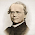## Wednesday, July 18, 2012

### Blacks and atheism

Razib Khan retweeted an invitation to join a live chat on how blacks don't do atheism. That made me wonder exactly what percent of American blacks don't believe in the existence of God. According to the GSS sample of 2,017 blacks, the 95% confidence interval is from 1.4 to 2.7%. For 11,866 whites, the interval is 2.4 to 3.0%. Since the two intervals overlap, there is no statistically significant racial difference.

UPDATE: Reader DR shows my error in the comments. Whites ARE significantly more likely than blacks to not believe in existence of God.

1.This post is technically incorrect on very basic statistics. You can't just compare the difference between two variables by seeing if their confidence interval overlaps.

Although means are additive, standard deviations, and hence confidence intervals are not. If you want a confidence interval for the difference between two random variables you have to construct a new standard deviation and apply it to the additive mean.

What we care about here is the difference between white, W, and black atheism, B levels, D= W - B. Specifically whether p(D > 0) > 95%. I.e. 95% confident (assuming no priors) that whites have higher levels of atheism then blacks.

Consulting wikipedia on computing the standard deviation of the difference between two estimators:

SD-E(Difference) = sqrt(SD-White^2 / NWhite + SD-Black^2 / NBlack)

Or more basically adding two standard deviations:

SD-E(Difference) = sqrt(SD-E(White)^2 + SD-E(Black)^2))

http://stattrek.com/estimation/difference-in-means.aspx

Assuming the confidence intervals you cite are using two sigma, or ~95%, then SD-E(White) = (SD-White/ NWhite)^2 = 0.15% and SD-E(Black) = (SD-Black / NBlack)^2 = 0.325%.

This yields an SD-E(Difference) of 0.3579%, applying that to the E(Difference) = 0.65% yields a two-sided two sigma confidence interval of [-0.0658%, 1.3658%].

However applying a two sided confidence interval is not correct in this case. Because the null hypothesis is not whether white-black atheism difference is less than 0. If your null hypothesis is an equality than you use a two sided test, but in this case it's a uni-directional inequality that we're rejecting.

So the correct sigma to use for 95% isn't 1.96, but 1.64. So the lower-bound confidence interval on the difference is 0.0613. Thus we can reject zero white-black atheism difference at the 95% confidence level.

You made some pretty basic mistakes in this post. I highly suggest you correct it. A lot of people interested and learning statistics read your blog. You don't want to give them misinformation about statistics methodology.

2.DR: Thanks--you're right. My mistake.

3.I remember watching the movie Fireproof, when the protagonist is sharing the gospel with a co-worker, and thinking, c'mon, he must have found the only black atheist fireman in the entire country.

Not entirely sure I followed the reasoning in the comment above, but for a normally distributed variable, but how did we get \sigma=1.64 for a .95 confidence interval?

### The Inductivist admits that culture matters

A pure naturist would claim that the culture of East Asians would not change after moving to America. Let's test this with one question ...